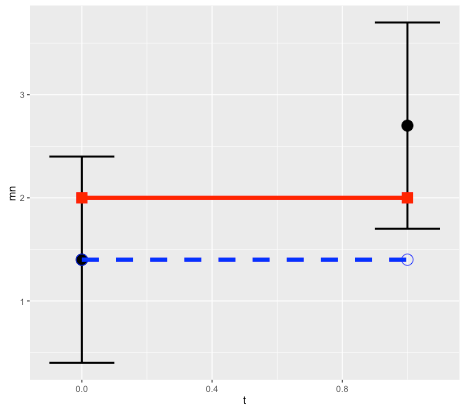# How do you calculate confidence intervals for a prediction?

This is a question that comes up frequently in forecasting. But it is surprisingly hard to answer, because it boils down to predicting how accurate a forecast will be – a prediction about a prediction. Prediction squared.

One approach is to base the estimate on past errors in similar situations. This method is used for example by the National  Institute of Statistics and Economic Studies (INSEE) in France, who wrote  that “the distribution of forecasting errors calculated from past exercises is a reliable indicator of the distribution of future errors and hence of the uncertainty  surrounding a given forecast” (see this research paper).

But this assumes that the new data will follow a familiar pattern – which may not be the case if for example you are trying to predict the effect of a novel economic policy, or a new drug, or climate change.

Another approach is to randomly perturb model parameters. But this has problems of its own.

To illustrate this, consider a simple linear model x(t) = k*t + x0, and suppose we want to predict the state at time t=1 based on an observation at t=0. Without loss of generality we can set the expected slope of the model to k=0, so the prediction is a persistence forecast: x1 = x0 (here x1 = x(1) and x0 = x(0)). Treating errors as random variables, then (in terms of variance) the error in the prediction, relative to the observation, will be the sum of the variance of the initial and final observational errors (see note below for details).Schematic for error in persistence model prediction. Black points are initial and final observations, with error bounds. Red line is true state (assumed to remain constant). Blue dashed line is prediction based on persistence model. Because the model is correct, all error is due to observations.

This makes sense since we are assuming the model is perfect, so all error comes from the observations. But in the real world, model error is not usually zero! Observational error is only part of the puzzle. So how do we estimate the contribution of model error?

As mentioned above, a typical approach is to perturb the parameters of the model by some reasonable amount, or do a Monte Carlo over a range of parameter values. (See paper on ensemble forecasting with model error.) For our simple linear model, a Monte Carlo simulation using a normal distribution around 0 with variance w for the parameter k would then give an ensemble of model predictions, with the same variance of w. Again this error will add to the error due to the observations.

This all sounds very logical and scientific, and versions of this approach are used by everyone from central bankers to weather forecasters. But again there is a catch, because the answer will depend on the parameter range that we selected. In other words, we can get whatever answer we want by choosing the range.

Of course, one can argue for a particular range – but if we are forecasting a new situation, we can’t base the estimate reliably on past data.

And there is an even more intractable issue – which is that the prediction error may be due not to parameter error, but to model structure. What if the actual system is not linear? (It probably isn’t.)

The ultimate problem is that the frequentist approach to statistics breaks down completely in forecasting – it relies on analyzing data, but the whole point of forecasting is that there is no data to measure (otherwise you could just measure it and not bother with the forecast).

Fortunately there is a solution, or at least an intellectually consistent method, which is to take a Bayesian approach. Unlike the commonly-taught frequentist approach, which treats probabilities as a measure of the frequency of observed events, the Bayesian approach interprets probabilities as a measure of degrees of belief. And in forecasting, confidence intervals ultimately are a measure of one’s confidence in the model.

In the case of our simple model, the idea is to come up with an initial confidence interval, based for example on previous experience, but see it as an estimate only, and refine it as more data comes in.

Of course this requires admitting that the confidence interval relies on subjective estimates. However doing so can help to avoid another problem in mathematical modelling, which is the tendency of frequentist error estimates to ignore the effect of context and prior information. Read our article on the BayesianOpinionator.

Notes:

For the simple linear model case, prediction error is the sum of the initial and final errors. We’ll use x to denote predictions, y for the true state, z for observations, and e for observational errors.

Suppose that the observed initial condition z0 is observed with an error e0, and the observed final point z1 has an error e1. So the true initial condition is y0 = z0 + e0, and the true final state is y1 = z1+ e1.

If we assume there is no model error, then y1 = y0. It follows that the difference between the forecast x1 = z0 and the observed final state z1 is:

error = z1- z0 = y1 – e1 – y0 + e0 = e0 – e1.

If the errors are assumed to be normal with variance v0 and v1, then the forecast error has variance v0 + v1 (variance is additive), which allows us to determine confidence intervals. So for example a 95% confidence interval would be +/-1.96 times the standard deviation.

If we assume that model error contributes an error at time 1 with variance w1, then again the variances are additive, and the total will increase to v0 + v1 + w1.